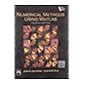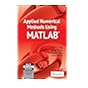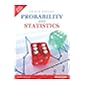Normal view

## Applied numerical methods using MATLAB / by Won Y. Yang, Wenwu Cao, Tae S. Chung and John Morris.

Material type:TextPublication details: New Delhi : J. Wiley, c2005Description: xiv, 508 p. : ill. ; 25 cmISBN: 9788126514151 (pbk)Subject(s): MATLAB | Numerical analysis -- Data processingDDC classification: 518
Contents:
1. MATLAB usage and computational errors -- 2. System of linear equations -- 3. Interpolation and curve fitting -- 4. Nonlinear equations -- 5. Numerical differentiation/integration -- 6. Ordinary differential equations -- 7. Optimization -- 8. Matrices and eigenvalues -- 9. Partial differential equations -- App. A. Mean value theorem -- App. B. Matrix operations/properties -- App. C. Differentiation with respect to a vector -- App. D. Laplace transform -- App. E. Fourier transform -- App. F. Useful formulas -- App. G. Symbolic computation -- App. H. Sparse matrices -- App. I. MATLAB.
Review: "Increasingly, scientists and engineers favor MATLAB over conventional programming languages, such as FORTRAN and C when they wish to solve complex problems. This book will enable readers to solve problems without needing to understand all the details of the underlying theory of numerical methods. By providing many examples of the uses of similar functions, it guides them towards the selection of the appropriate MATLAB functions for solving their problem efficiently."--BOOK JACKET.
Tags from this library: No tags from this library for this title.Average rating: 0.0 (0 votes)
Item type Current library Call number Status Date due Barcode Item holdsBooks Namal Library
Mathematics
518 YAN-A 2005 3321 (Browse shelf (Opens below)) Available 0003321
Total holds: 0
##### Browsing Namal Library shelves, Shelving location: Mathematics Close shelf browser (Hides shelf browser)518 MAT-N 2004 3384 Numerical methods using MATLAB / 518 PAL-D 2012 3884 Differential equations, mechanics, and computation / 518 POL-O 2009 3755 Ordinary differential equations using MATLAB / 518 YAN-A 2005 3321 Applied numerical methods using MATLAB / 519 DAS-A 2016 10924 Applied mathematics for polytechnics/ 519 DAS-A 2016 10925 Applied mathematics for polytechnics/ 519.024 DEG-P 2017 9736 Probability and statistics /

Includes bibliographical references and index.

1. MATLAB usage and computational errors -- 2. System of linear equations -- 3. Interpolation and curve fitting -- 4. Nonlinear equations -- 5. Numerical differentiation/integration -- 6. Ordinary differential equations -- 7. Optimization -- 8. Matrices and eigenvalues -- 9. Partial differential equations -- App. A. Mean value theorem -- App. B. Matrix operations/properties -- App. C. Differentiation with respect to a vector -- App. D. Laplace transform -- App. E. Fourier transform -- App. F. Useful formulas -- App. G. Symbolic computation -- App. H. Sparse matrices -- App. I. MATLAB.

"Increasingly, scientists and engineers favor MATLAB over conventional programming languages, such as FORTRAN and C when they wish to solve complex problems. This book will enable readers to solve problems without needing to understand all the details of the underlying theory of numerical methods. By providing many examples of the uses of similar functions, it guides them towards the selection of the appropriate MATLAB functions for solving their problem efficiently."--BOOK JACKET.

There are no comments on this title.